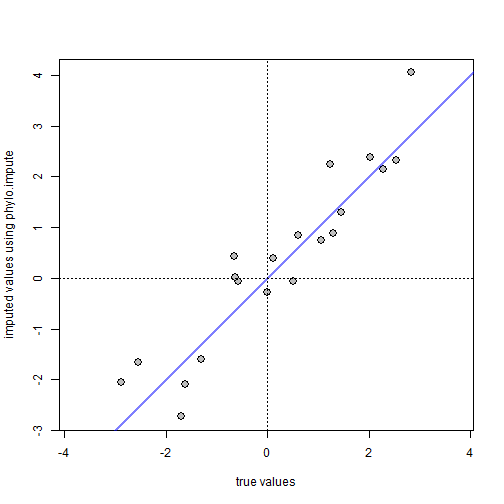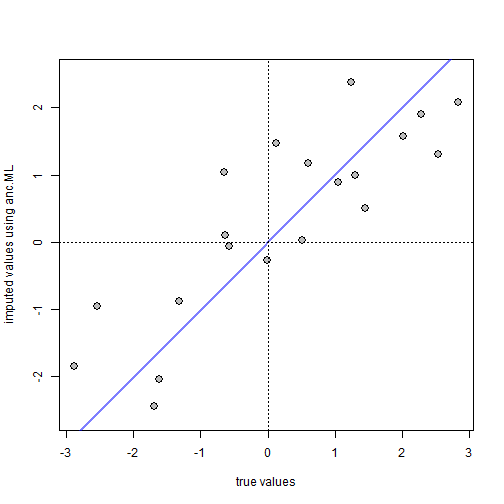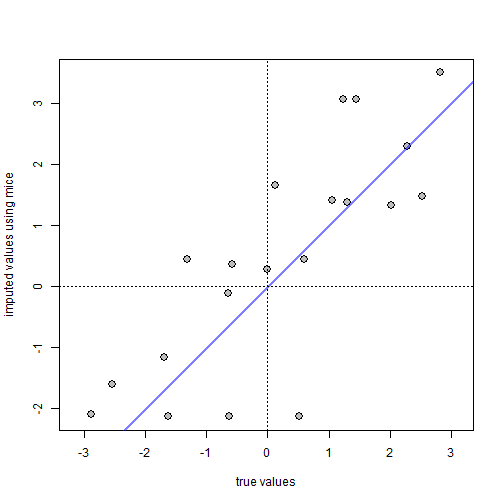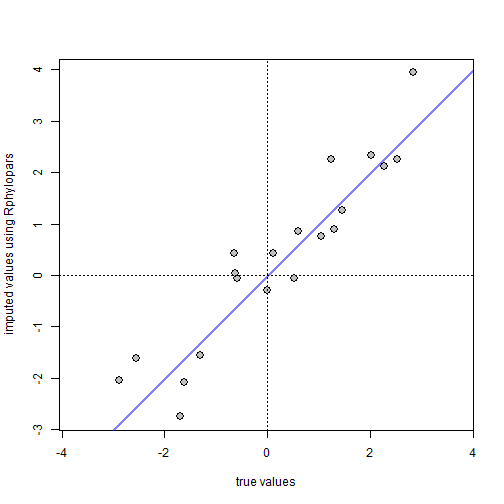## Thursday, March 7, 2019

### Phylogenetic imputation with multivariate data

A colleague recently asked me about the question of phylogenetic imputation - that is, the substitution of estimated values (from some procedure) for missing items in a data matrix.

Actually, phytools already has a function to do just this for a single variable in the form of `anc.ML`. In this case if any species in the tree are missing from the input vector, what the function does is estimate these missing values jointly with ancestral states using Maximum Likelihood.

However, in the case of multiple correlated continuous traits we ought to be able to do much better since we can take both the correlation structure implied by the tree and that implied by the covariances among characters into account simultaneously.

Now, before I go any further, I should mention that it turns out to be the case that the CRAN package Rphylopars (by Eric Goolsby, Jorn Bruggeman, and Cécile Ané) already effectively does just that, but before knowing that I had already written my own phylogenetic imputation function, and as it is still slightly different from Rphylopars I thought I'd share it just the same.

Here is the function (& associated internals & methods):

``````expand.range<-function(x,na.rm=FALSE,p=2.0){
rr<-range(x,na.rm=na.rm)
mean(rr)+c(p*(rr-mean(rr)),p*(rr-mean(rr)))
}

phylo.impute<-function(tree,X,...){
if(hasArg(maxit)) maxit<-list(...)\$maxit
else maxit<-5000
if(hasArg(quiet)) quiet<-list(...)\$quiet
else quiet<-TRUE
if(hasArg(fixed)) fixed<-list(...)\$fixed
else fixed<-FALSE
if(hasArg(p)) p<-list(...)\$p
else p<-2.0
if(is.data.frame(X)) X<-as.matrix(X)
ii<-which(is.na(X),arr.ind=TRUE)
lik<-function(theta,tree,X,ii){
X[ii]<-theta
object<-evol.vcv(tree,X)
if(!quiet) print(object\$logL1)
-object\$logL1
}
lower<-apply(X,2,expand.range,na.rm=TRUE,p=p)[1,ii[,2]]
upper<-apply(X,2,expand.range,na.rm=TRUE,p=p)[2,ii[,2]]
if(hasArg(x.init)) x.init<-list(...)\$x.init
else x.init<-"ace"
if(x.init=="random") x.init<-runif(n=length(ii),lower,upper)
else if(x.init=="ace") {
x.init<-vector()
for (i in 1:nrow(ii)){
tip<-rownames(X)[ii[i]]
tt<-drop.tip(root(tree,outgroup=tip),
names(ii[which(ii[,2]==ii[i,2]),1]))
x.init[i]<-fastAnc(tt,X[!is.na(X[,ii[i,2]]),
ii[i,2]])
}
}
if(fixed==FALSE){
fit<-optim(x.init,lik,tree=tree,X=X,ii=ii,method="L-BFGS-B",
lower=lower,upper=upper,control=list(maxit=maxit))
} else {
fit<-list(par=x.init,value=lik(x.init,tree,X,ii),
counts=c(0,0),convergence=0,
message="Fixed value of par.")
}
X[ii]<-fit\$par
attr(X,"optim")<-list(logLik=-fit\$value,counts=fit\$counts,
convergence=fit\$convergence,message=fit\$message)
class(X)<-c("matrix","phylo.impute")
X
}

print.phylo.impute<-function(x,...){
if(hasArg(digits)) digits<-list(...)\$digits
else digits<-c(7,3)
if(hasArg(n)) n<-list(...)\$n
else n<-6L
if(n>nrow(x)) n<-nrow(x)
cat("Results from phylogenetic imputation:\n")
if(n<nrow(x)) cat("...\n\n") else cat("\n")
cat(paste("log(L) :",round(attr(x,"optim")\$logLik,digits),"\n"))
if(attr(x,"optim")\$convergence==0)
cat("R thinks it has converged on the correct solution.\n\n")
else
cat("R thinks it may not have converged.\n\n")
}
``````

As way of explanation, the function uses `phytools::evol.vcv` internally to compute the likelihood. What it is thus doing is maximizing the likelihood of a set of missing tip values and the parameters of the fitted multivariate BM model, which has an expected multivariate normal density that is the Kronecker (⊗) product of the among-species covariance matrix implied by the tree and the trait variance-covariance matrix.

Let's test it out with some simulated data. Here, I'll generate a set of three characters with some correlation, then I'll randomly remove some fraction of items:

``````library(phytools)
tree<-pbtree(n=40)
V<-matrix(c(1,0.8,-0.4,
0.8,1,-0.3,
-0.4,-0.3,1),3,3)
V
``````
``````##      [,1] [,2] [,3]
## [1,]  1.0  0.8 -0.4
## [2,]  0.8  1.0 -0.3
## [3,] -0.4 -0.3  1.0
``````
``````X.full<-sim.corrs(tree,V)
X.missing<-X.full
X.missing[cbind(sample(1:nrow(X.missing),20),
sample(1:3,20,replace=TRUE))]<-NA
X.missing
``````
``````##           [,1]        [,2]       [,3]
## t28  0.8886526  1.24411355 -0.4245481
## t29  0.5247258          NA -0.3488527
## t15  1.0991001  0.93130587         NA
## t7          NA -0.10577827  0.9283790
## t8   1.6537325  0.63667970 -1.6943908
## t3   0.9411518          NA  1.9152188
## t12         NA  1.05780716  0.6967641
## t13  3.0608484  1.17402077 -0.7259395
## t6          NA  2.96681644 -0.5676536
## t11  0.5996629  0.88238169 -1.2939165
## t33  0.8396649  1.11687510 -1.6444932
## t34  0.9295234  1.31738154 -2.0104121
## t14 -0.5887746 -0.12207405 -0.9916148
## t4   2.3153214          NA -2.3048569
## t39  1.3675492          NA -1.2902536
## t40  1.0410807  1.89692359 -1.1669341
## t26         NA  2.02956710 -2.4545838
## t27  0.9084507  2.28359305 -2.4078693
## t16  3.1116469  2.97597913         NA
## t17  3.2297573  2.34094985 -2.5940189
## t19  2.5269104  2.23501573 -3.1675657
## t20  1.4105685  1.60584519 -2.6611642
## t9          NA  1.47446649 -0.2450809
## t10 -0.7050291 -0.25625707 -0.1703774
## t24  1.6559010  0.04879502 -1.4309771
## t25         NA  0.94049426 -1.1473610
## t5   1.3972257  0.43433575  0.5023487
## t35  1.2134691  0.48637670  0.2816280
## t36  0.4015474 -0.24977225  0.6174954
## t21  1.2407177  0.02087955         NA
## t18  1.2346912  0.35923112         NA
## t2   1.4738868  0.05407450 -2.0972510
## t31  0.0616486 -1.05296533         NA
## t32 -0.7800648          NA -1.8888676
## t37         NA -0.17341178 -2.1374960
## t38  0.8885034 -0.01562105         NA
## t30  0.8538446          NA -1.6322041
## t22  1.3147619  0.96365117 -1.6031923
## t23  1.2779305  0.75501629 -1.7627702
## t1   3.4946181  1.81298955         NA
``````

Now, let's try to impute the missing values using `phylo.impute`:

``````X.imputed<-phylo.impute(tree,X.missing)
X.imputed
``````
``````## Results from phylogenetic imputation:
##          [,1]       [,2]       [,3]
## t28 0.8886526  1.2441135 -0.4245481
## t29 0.5247258  0.8422554 -0.3488527
## t15 1.0991001  0.9313059 -0.2878059
## t7  0.3810040 -0.1057783  0.9283790
## t8  1.6537325  0.6366797 -1.6943908
## t3  0.9411518  0.4394695  1.9152188
## ...
##
## log(L) : -106.429
## R thinks it has converged on the correct solution.
``````

Now, let's compare the true missing item values to their imputed values using the method:

``````missing<-which(is.na(X.missing),arr.ind=TRUE)
plot(X.full[missing],X.imputed[missing],pch=21,bg="grey",cex=1.5,
xlab="true values",ylab="imputed values using phylo.impute",asp=1)
lines(x=c(min(par()\$usr[c(1,3)]),max(par()\$usr[c(2,4)])),
y=c(min(par()\$usr[c(1,3)]),max(par()\$usr[c(2,4)])),
col=make.transparent("blue",0.5),lwd=2)
abline(h=0,lty="dotted")
abline(v=0,lty="dotted")
````````````cor(X.full[missing],X.imputed[missing])
``````
``````##  0.9332328
``````

This looks pretty good, but is hard to evaluate unless we compare it to other methods. The two obvious choices are: (1) using the phylogeny but not the other traits; and (2) using the other traits but not the phylogeny. The former, as I already mentioned, we can do using `phytools::anc.ML` as follows:

``````x1<-X.missing[,1]
fit1<-anc.ML(tree,x1[!is.na(x1)])
x2<-X.missing[,2]
fit2<-anc.ML(tree,x2[!is.na(x2)])
x3<-X.missing[,3]
fit3<-anc.ML(tree,x3[!is.na(x3)])
X.ace<-X.missing
X.ace[names(fit1\$missing.x),1]<-fit1\$missing.x
X.ace[names(fit2\$missing.x),2]<-fit2\$missing.x
X.ace[names(fit3\$missing.x),3]<-fit3\$missing.x
plot(X.full[missing],X.ace[missing],pch=21,bg="grey",cex=1.5,
xlab="true values",ylab="imputed values using anc.ML",asp=1)
lines(x=c(min(par()\$usr[c(1,3)]),max(par()\$usr[c(2,4)])),
y=c(min(par()\$usr[c(1,3)]),max(par()\$usr[c(2,4)])),
col=make.transparent("blue",0.5),lwd=2)
abline(h=0,lty="dotted")
abline(v=0,lty="dotted")
````````````cor(X.full[missing],X.ace[missing])
``````
``````##  0.8478251
``````

Imputation without the phylogeny but using the correlation structure of the traits has many different flavors. I decided to use a sophisticated approach from the R package mice, assuming that simpler approaches would not do better:

``````library(mice)
X.mice<-as.matrix(complete(mice(X.missing)))
``````
``````##
##  iter imp variable
##   1   1  V1  V2  V3
##   1   2  V1  V2  V3
##   1   3  V1  V2  V3
##   1   4  V1  V2  V3
##   1   5  V1  V2  V3
##   2   1  V1  V2  V3
##   2   2  V1  V2  V3
##   2   3  V1  V2  V3
##   2   4  V1  V2  V3
##   2   5  V1  V2  V3
##   3   1  V1  V2  V3
##   3   2  V1  V2  V3
##   3   3  V1  V2  V3
##   3   4  V1  V2  V3
##   3   5  V1  V2  V3
##   4   1  V1  V2  V3
##   4   2  V1  V2  V3
##   4   3  V1  V2  V3
##   4   4  V1  V2  V3
##   4   5  V1  V2  V3
##   5   1  V1  V2  V3
##   5   2  V1  V2  V3
##   5   3  V1  V2  V3
##   5   4  V1  V2  V3
##   5   5  V1  V2  V3
``````
``````plot(X.full[missing],X.mice[missing],pch=21,bg="grey",cex=1.5,
xlab="true values",ylab="imputed values using mice",asp=1)
lines(x=c(min(par()\$usr[c(1,3)]),max(par()\$usr[c(2,4)])),
y=c(min(par()\$usr[c(1,3)]),max(par()\$usr[c(2,4)])),
col=make.transparent("blue",0.5),lwd=2)
abline(h=0,lty="dotted")
abline(v=0,lty="dotted")
````````````cor(X.full[missing],X.mice[missing])
``````
``````##  0.7944393
``````

Finally, we can compare to Rphylopars. Here, there is basically no difference (although it's evident that the functions are doing slightly different things as the values do not match exactly), except for the important difference that Rphylopars is much faster:

``````X.phylopars<-as.data.frame(X.missing)
X.phylopars<-cbind(species=rownames(X.phylopars),X.phylopars)
object<-phylopars(X.phylopars,tree)
plot(X.full[missing],object\$anc_recon[missing],pch=21,bg="grey",cex=1.5,
xlab="true values",ylab="imputed values using Rphylopars",asp=1)
lines(x=c(min(par()\$usr[c(1,3)]),max(par()\$usr[c(2,4)])),
y=c(min(par()\$usr[c(1,3)]),max(par()\$usr[c(2,4)])),
col=make.transparent("blue",0.5),lwd=2)
abline(h=0,lty="dotted")
abline(v=0,lty="dotted")
````````````cor(X.full[missing],object\$anc_recon[missing])
``````
``````##  0.9318714
``````

That's all. In summary, I'd recommend using Rphylopars for any more than a handful of imputed values as otherwise the likelihood optimization of my code becomes very time consuming.

1.hi Liam,

thanks for sharing this post. there are more R packages providing 'phylogenetic imputation' but all of them are designed to handle continuous data only. however, is there any method of phylogenetic imputation for categorical/discrete traits?

thanks!

gabriel

2.Hi Liam,

I second Gabriel's comments. Do you know of a phylogenetic imputation method for binary characters?

Thanks
VInny

Note: due to the very large amount of spam, all comments are now automatically submitted for moderation.